Courses

# Test: Correlation And Regression- 6

## 40 Questions MCQ Test Business Mathematics and Logical Reasoning & Statistics | Test: Correlation And Regression- 6

Description
This mock test of Test: Correlation And Regression- 6 for CA Foundation helps you for every CA Foundation entrance exam. This contains 40 Multiple Choice Questions for CA Foundation Test: Correlation And Regression- 6 (mcq) to study with solutions a complete question bank. The solved questions answers in this Test: Correlation And Regression- 6 quiz give you a good mix of easy questions and tough questions. CA Foundation students definitely take this Test: Correlation And Regression- 6 exercise for a better result in the exam. You can find other Test: Correlation And Regression- 6 extra questions, long questions & short questions for CA Foundation on EduRev as well by searching above.
QUESTION: 1

Solution:
QUESTION: 2

Solution:
QUESTION: 3

### Given the following data: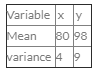Coefficient of correlation = 0.6 What is the most likely value of y when x = 90?

Solution:
QUESTION: 4

If high values of one tend to low values of the other, they are said to be

Solution:
QUESTION: 5

___________ is concerned with the measurement of the “strength of association” between variables.

Solution:
QUESTION: 6

The two lines of regression are given by
8x+10y=25 and 16x+5y=12 respectively
If the variance of x is 25, what is the standard deviation of y?

Solution:
QUESTION: 7

The regression equation of y on x for the following data: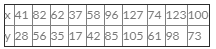Is given by

Solution:
QUESTION: 8

The two regression coefficients for the following data: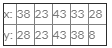are

Solution:
QUESTION: 9

The coefficient of correlation between cost of advertisement and sales of a product on the basis of the following data: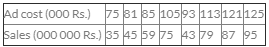is:

Solution:
QUESTION: 10

_________ gives the mathematical relationship of the variables.

Solution:
QUESTION: 11

What is the coefficient of concurrent deviations for the following data: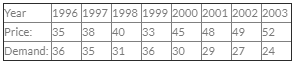Solution:
QUESTION: 12

If y=3x+4 is the regression line of y on x and the arithmetic mean of x is -1, what is the arithmetic mean of y?

Solution:
QUESTION: 13

Given that for twenty pairs of observations, ?xu=525, ?x=129, ?u=97, ?x2=687, ?u2=427 and y=10-3u, the coefficient of correlation between x and y is

Solution:
QUESTION: 14

What is the coefficient of correlation between the ages of husbands and wives from the following data?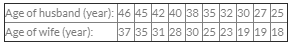Solution:
QUESTION: 15

The following results relate to bivariate date on (x, y):
Σxy=414, ∑x=120, ∑y=90, ∑x2=600, ∑y2=300, n=30. Later or, it was known that two pairs of observations (12, 11) and (6, 8) were wrongly taken, the correct pairs of observations being (10,9) and (8, 10). The corrected value of the correlation coefficient is

Solution:
QUESTION: 16

What is the value of Rank correlation coefficient between the following marks in physics and Chemistry: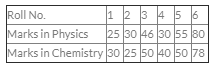Solution:
QUESTION: 17

Eight contestants in a musical contest were ranked by two judges A and B in the following manner: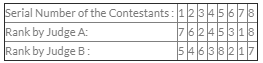The rank correlation coefficient

Solution:
QUESTION: 18

The following table provides the distribution of items according to size groups and also the number of defectives: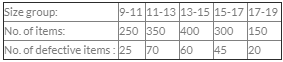The correlation coefficient between size and defectives is

Solution:
QUESTION: 19

If u = 2x+5 and v=-3y-6 and regression coefficient of y on x is 2.4, what is the regression coefficient of v on u?

Solution:
QUESTION: 20

What is the coefficient of correlation from the following data?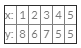Solution:
QUESTION: 21

Following are the marks of 10 students in Botany and Zoology: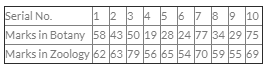The coefficient of rank correlation between marks in Botany and Zoology is

Solution:
QUESTION: 22

If 4y-5x=15 is the regression line of y on x and the coefficient of correlation between x and y is 0.75, what is the value of the regression coefficient of x on y?

Solution:
QUESTION: 23

For two variables x and y, it is known that cov (x, y)=80, variance of x is 16 and sum of squares of deviation of y from its mean is 250. The number of observations for this bivariate data is

Solution:

Solution :- Covariance between  X  and  Y  is:  C(X,Y)=80

Variance of  X  is:  V(X)=16

Variance of  Y  is:  V(Y)=E(Y−E(Y))^2=250/n

The Coefficient of correlation between  X  and  Y  is:

ρ(X,Y)=C(X,Y)/[√V(X)√V(Y)

= 80/√16*√250/n

=80√n/(√16*√250)

=√=80n/20*√10)

=4√n/10

Check that  4√n10 > 1  ∀n∈N,but the coefficient of correlation must always be less than or equal to 1.

QUESTION: 24

The coefficient of correlation between x and y where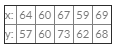is:

Solution:
QUESTION: 25

f(x) = x2/ethen f’(1) is equal to _________.

Solution:
QUESTION: 26

If f(x) = xk and f’(1) = 10 the value of k is

Solution:
QUESTION: 27

If f(x) =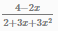then the values of x for which f’(x) = 0 is

Solution:
QUESTION: 28

If  y=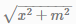then y y1 (where y= dy/dx) is equal to

Solution:
QUESTION: 29

If y = e+ e-x then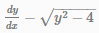is equal to

Solution:
QUESTION: 30

Given f(x) = 4x+ 3x- 2x + 5 and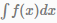is

Solution:
QUESTION: 31

If the covariance between two variables is 20 and the variance of one of the variables is 16, what would be the variance of the other variable?

Solution:
QUESTION: 32

What are the limits of the two regression coefficients?

Solution:
QUESTION: 33

If the coefficient of correlation between two variables is 0.7 then the percentage of variation unaccounted for is

Solution:

Step-by-step explanation:

Given:  the coefficient of correlation between two variables is 0.7.

then the percentage of variation accounted for is given by

= square of coefficient of correlation

=  0.7^2 = 0.49

then the percentage of variation unaccounted for is = 1-0.49 =0.51

hence option c is correct

QUESTION: 34

If y = a+bx, then what is the coefficient of correlation between x and y?

Solution:
QUESTION: 35

If there are two variables x and y, then the number of regression equations could be

Solution:
QUESTION: 36

If cov(x, y) = 15, what restrictions should be put for the standard deviations of x and y?

Solution:
QUESTION: 37

The regression coefficient remain unchanged due to a

Solution:
QUESTION: 38

The regression line of y on is derived by

Solution:
QUESTION: 39

If the coefficient of correlation between two variables is -0.9, then the coefficient of determination is

Solution:
QUESTION: 40

The two lines regression become identical when

Solution: